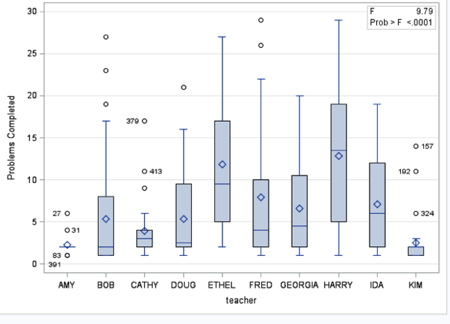# What makes the difference in a classroom?

A couple of weeks ago, I wrote a post that showed student achievement was not what made a difference in whether students persisted in playing our games. With the exception of a few students who were very low achieving, e.g., non-readers in fourth-grade and up, there was little difference in the pretest scores of students who gave up at the first math problem and those who persisted.

What did make the difference, then? My next hypothesis was that it was the teacher that made a difference. To test this hypothesis, I did an Analysis of Variance with SAS using PROC GLM. The WHERE statement was used to eliminate extreme observations from the analysis – students who had attempted more than 50 problems. Complete code is shown below.

`PROC GLM DATA= teach ;`

`CLASS teacher ;`

`MODEL prob_correct_N = teacher ;`
``` LABEL prob_correct_N = “Problems Completed” ;```

`WHERE prob_correct_N < 50 ;`

The teacher variable explained 22% of the variance (F = 9.79, p < .0001). When grade level (grades 4-7 were included in the analysis) was added to the model, the additional explained variance was trivial – about 0.5%.In the figure above, produced by default for this analysis when ODS GRAPHICS is on, a box and whisker plot is given for each teacher, showing mean, median and outliers (note the numbers on the plot are observation number for outliers and not a value for the dependent variable).  There are clear differences among the teachers in mean number of problems completed.

The next step (thank goodness this is a longitudinal study) is to see what those teachers are doing that explains persistence.

Shameless plug #1 :

I will be giving a talk at the Western Users of SAS Software conference tomorrow (Thursday, September 8, 2016) at the Grand Hyatt in San Francisco that includes a lot more detail about the use of SAS for program evaluation.

Shameless plug # 2:

You can buy the games that are the basis of these data here. Fun and educational. Run on Mac or Windows.